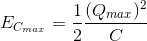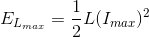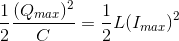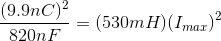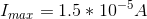# AP Physics C Electricity : Understanding Electrical-Magnetic Equivalents

## Example Questions

### Example Question #1 : Electromagnetism

A capacitor and an inductor mH are connected in series to complete a simple circuit. The capacitor is initially charged to 9.9 nC.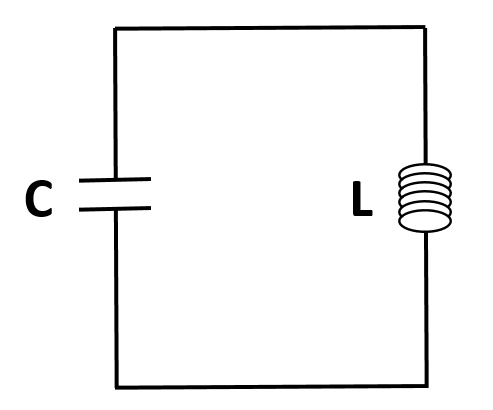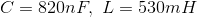What is the value of the maximum current that passes through the circuit?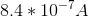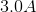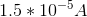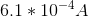Explanation:

Since there are no dissipative circuit elements (such as a resistor) present in the circuit, the energy originally held by the electric field of the capacitor oscillates between electric energy and magnetic energy over time. As such, the maximum electric energy of the capacitor is equal in value to the maximum magnetic energy of the inductor.